# 0.8 Exponential functions and graphs

 Page 1 / 2

## Introduction

In Grade 10, you studied graphs of many different forms. In this chapter, you will learn a little more about the graphs of exponential functions.

## Functions of the form $y=a{b}^{\left(x+p\right)}+q$ For $b>0$

This form of the exponential function is slightly more complex than the form studied in Grade 10.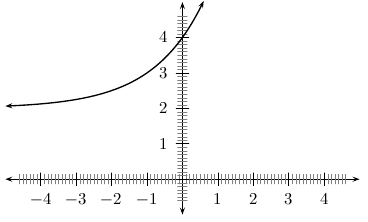General shape and position of the graph of a function of the form f ( x ) = a b ( x + p ) + q .

## Investigation : functions of the form $y=a{b}^{\left(x+p\right)}+q$

1. On the same set of axes, with $-5\le x\le 3$ and $-35\le y\le 35$ , plot the following graphs:
1. $f\left(x\right)=-2·{2}^{\left(x+1\right)}+1$
2. $g\left(x\right)=-1·{2}^{\left(x+1\right)}+1$
3. $h\left(x\right)=0·{2}^{\left(x+1\right)}+1$
4. $j\left(x\right)=1·{2}^{\left(x+1\right)}+1$
5. $k\left(x\right)=2·{2}^{\left(x+1\right)}+1$
Use your results to understand what happens when you change the value of $a$ . You should find that the value of $a$ affects whether the graph curves upwards ( $a>0$ ) or curves downwards ( $a<0$ ). You should also find that a larger value of $a$ (when $a$ is positive) stretches the graph upwards. However, when $a$ is negative, a lower value of $a$ (such as -2 instead of -1) stretches the graph downwards. Finally, note that when $a=0$ the graph is simply a horizontal line. This is why we set $a\ne 0$ in the original definition of these functions.
2. On the same set of axes, with $-3\le x\le 3$ and $-5\le y\le 20$ , plot the following graphs:
1. $f\left(x\right)=1·{2}^{\left(x+1\right)}-2$
2. $g\left(x\right)=1·{2}^{\left(x+1\right)}-1$
3. $h\left(x\right)=1·{2}^{\left(x+1\right)}+0$
4. $j\left(x\right)=1·{2}^{\left(x+1\right)}+1$
5. $k\left(x\right)=1·{2}^{\left(x+1\right)}+2$
Use your results to understand what happens when you change the value of $q$ . You should find that when $q$ is increased, the whole graph is translated (moved) upwards. When $q$ is decreased (poosibly even made negative), the graph is translated downwards.
3. On the same set of axes, with $-5\le x\le 3$ and $-35\le y\le 35$ , plot the following graphs:
1. $f\left(x\right)=-2·{2}^{\left(x+1\right)}+1$
2. $g\left(x\right)=-1·{2}^{\left(x+1\right)}+1$
3. $h\left(x\right)=0·{2}^{\left(x+1\right)}+1$
4. $j\left(x\right)=1·{2}^{\left(x+1\right)}+1$
5. $k\left(x\right)=2·{2}^{\left(x+1\right)}+1$
Use your results to understand what happens when you change the value of $a$ . You should find that the value of $a$ affects whether the graph curves upwards ( $a>0$ ) or curves downwards ( $a<0$ ). You should also find that a larger value of $a$ (when $a$ is positive) stretches the graph upwards. However, when $a$ is negative, a lower value of $a$ (such as -2 instead of -1) stretches the graph downwards. Finally, note that when $a=0$ the graph is simply a horizontal line. This is why we set $a\ne 0$ in the original definition of these functions.
4. Following the general method of the above activities, choose your own values of $a$ and $q$ to plot 5 graphs of $y=a{b}^{\left(x+p\right)}+q$ on the same set of axes (choose your own limits for $x$ and $y$ carefully). Make sure that you use the same values of $a$ , $b$ and $q$ for each graph, and different values of $p$ . Use your results to understand the effect of changing the value of $p$ .

These different properties are summarised in [link] .

 $p<0$ $p>0$ $a>0$ $a<0$ $a>0$ $a<0$ $q>0$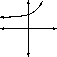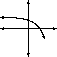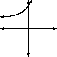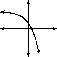$q<0$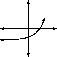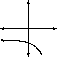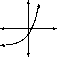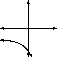## Domain and range

For $y=a{b}^{\left(x+p\right)}+q$ , the function is defined for all real values of $x$ . Therefore, the domain is $\left\{x:x\in \mathbb{R}\right\}$ .

The range of $y=a{b}^{\left(x+p\right)}+q$ is dependent on the sign of $a$ .

If $a>0$ then:

$\begin{array}{ccc}\hfill {b}^{\left(x+p\right)}& >& 0\hfill \\ \hfill a·{b}^{\left(x+p\right)}& >& 0\hfill \\ \hfill a·{b}^{\left(x+p\right)}+q& >& q\hfill \\ \hfill f\left(x\right)& >& q\hfill \end{array}$

Therefore, if $a>0$ , then the range is $\left\{f\left(x\right):f\left(x\right)\in \left[q,\infty \right)\right\}$ . In other words $f\left(x\right)$ can be any real number greater than $q$ .

If $a<0$ then:

$\begin{array}{ccc}\hfill {b}^{\left(x+p\right)}& >& 0\hfill \\ \hfill a·{b}^{\left(x+p\right)}& <& 0\hfill \\ \hfill a·{b}^{\left(x+p\right)}+q& <& q\hfill \\ \hfill f\left(x\right)& <& q\hfill \end{array}$

what are the importance of studying economics
To know if the country is growing or not through the country's GDP
Ariel
What is the law of demand
price increase demand decrease...price decrease demand increase
Mujahid
ıf the price increase the demand decrease and if the demand increase the price decrease
MUBARAK
all other things being equal, an increase in demand causes a decrease in supply and vice versa
SETHUAH
yah
Johnson
how is the economy of usa now
Johnson
What is demand
Demand is the quantity of goods and services a consumer is willing and able to purchase at various prices over a given period of time.
Yaw
yea
SETHUAH
Okay congratulations I'll join you guys later .
Aj
yes
MUBARAK
calculate elasticity of income exercises
If potatoes cost Jane $1 per kilogram and she has$5 that could possibly spend on potatoes or other items. If she feels that the first kilogram of potatoes is worth $1.50, the second kilogram is worth$1.14, the third is worth $1.05 and subsequent kilograms are worth$0.30, how many kilograms of potatoes will she purchase? What if she only had $2 to spend? Susan Reply cause of poverty in urban DAVY Reply QI: (A) Asume the following cost data are for a purely competitive producer: At a product price Of$56. will this firm produce in the short run? Why Why not? If it is preferable to produce, what will be the profit-maximizing Or loss-minimizing Output? Explain. What economic profit or loss will the
what is money
money is any asset that is generally acceptable for the exchange of goods and for the settlement of debts
Mnoko
what is economic
economics is the study of ways in which people use resources to satisfy their wants
Falak
what is Price mechanism
what is Economics
ERNESTINA
The study of resource allocation,distribution and consumption.
Emelyn
introduction to economics
welfare definition of economics
Uday
examine the wealth and welfare definitions of economics
Uday
Anand
What do we mean by Asian tigers
Dm me I will tell u
Shailendra
Hi
Aeesha
hi
Pixel
What is Average revenue
KEMZO
How are u doing
KEMZO
it is so fantastic
metasebia
uday
Uday
it is a group of 4 countries named Singapore, South Korea, Taiwan and Hong Kong because their economies are growing very faster
Anand
fyn
EDWARD
Please, average revenue is an amount of money you gained after deducted your total expenditure from your total income.
EDWARD
what's a demand
it is the quantity of commodities that consumers are willing and able to purchase at particular prices and at a given time
Munanag
quantity of commodities dgat consumers are willing to pat at particular price
Omed
demand depends upon 2 things 1wish to buy 2 have purchasing power of that deserving commodity except any from both can't be said demand.
Bashir
Demand is a various quantity of a commodities that a consumer is willing and able to buy at a particular price within a given period of time. All other things been equal.
Vedzi
State the law of demand
Vedzi
The desire to get something is called demand.
Mahabuba
what is the use of something should pay for its opportunity foregone to indicate?
Why in monopoly does the firm maximize profits when its marginal revenue equals marginal cost
Researchers demonstrated that the hippocampus functions in memory processing by creating lesions in the hippocampi of rats, which resulted in ________.
The formulation of new memories is sometimes called ________, and the process of bringing up old memories is called ________.
Other chapter Q/A we can ask

#### Get Jobilize Job Search Mobile App in your pocket Now!By Anh DaoBy Stephen VoronBy Anonymous UserBy Yacoub JayoghliBy Abby SharpBy OpenStaxBy OpenStaxBy OpenStaxBy Jesenia WoffordBy Brooke Delaney